# Mass + system of equations - math problems

#### Number of problems found: 79

• Copper partsFrom copper cast were made 3 parts. At first consumed one third of casting, the second 2/3 of rest and the third weighed 18 kg. What is the weight of original copper cast?
• ConcentrationHow many grams of 65% and 50% acid is to be mixed to give 240 g acid with a concentration of 60%?
• Two melons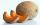There are 2 melons in the box. Together they weigh 11kg. The weight of green is 3 kg less than three times the weight of yellow. What is the weight of a green melon?
• Three granaries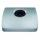Three granaries hold 7,800 metric cent (q) of grain. In the first, 70 tonnes of grain was more than the second and in the third it was 120 tonnes less than in the second. How many grain were in each granary?
• Bags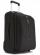The first bag has a weight of 18 kg, the second bag is a one-third lighter than the first and the third bag has three times less the weight of all three bags together. Determine their weights.
• CoinsThe money - coins are minted from the hardest bronze, which contains copper and tin in a ratio of 41: 9. How much copper and tin are in 2kg of bronze money?
• Cargo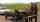Weight of cargo car is 761 kg. Weight of car is 23% of the total weight of car+cargo. What is the weight of the cargo load?
• The water barrel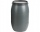The water barrel weighs 122 kg. If we pour 75% of the water out of it, it will weigh 35 kg. What is the weight of the barrel?
• Crates 2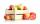In 6 crates is 45 kg of apples In  5 crates is equally In 1 in crate is 3 kg more How many kilograms in each crate?
• Reservoir + waterReservoir completely filled with water weighs 12 kg. After pouring off three quarters of the amount of water weights 3 kg. Calculate the weight and volume of the reservoir.
• BonbonsCreate a mixture of 50 kg of candy on price 700Kč. Candies has prices: 820Kč, 660Kč and 580Kč. Use cross rule.
• Coal storage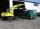The coal storage distribute received coal shipment within three days. The first day distribute third of the shipments, the second day of two-fifths of the rest and the third day 300 tons of coal. How many tons of coal distributed first and second day?
• Warehouses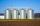In the three warehouses, a total of 70 tons of grain was stored. In the second warehouse was stored 8.5t less and in the third 3.5t more than in the first. How many tons of grain was stored in each warehouse?
• PotatoesFor three days the store sold 1400 kg of potatoes. The first day they sold 100 kilograms of potatoes less than the second day, the third-day three-fifths of what they sold the first day. How many kgs of potatoes sold every day?
• Three friendsDanica, Lenka and Dalibor have altogether 96 kg. Lenka weighs 75% more than Dalibor and Danica weighs 6 kg more than Dalibor. Determine the weight of Danice, Lenka and Dalibor.
• Water containerContainer with water weighs 1.48 kg. When we cast 75% of water container of water weight 0.73 kg. How heavy is an empty container?
• Barrel with liquid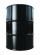Barrel with grain weight 297 kg. When it shed 48% of grain, ha weight 174 kg. What is the weight of empty barrel?
• Buns and toastsTwo buns weigh 10 grams more than two toasts. One Bun and two Toast weigh a total of 110 grams. How many grams weigh three toasts? How many grams does a Bun weigh?
• Barrel 4Barrel of water weighs 63 kg. After off 75% water, the weight of the barrel with water is 21 kg. How many kg weigh empty barrel and how many kgs water in it?
• Trio weight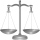Adelka, Barunka, and Cecilka are weight in pairs. Adelka with Barunka weighs 98 kg, Barunka with Cecilka 90 kg and Adelka with Cecilka 92 kg. How much does each of them weigh?

Do you have an interesting mathematical word problem that you can't solve it? Submit a math problem, and we can try to solve it.

We will send a solution to your e-mail address. Solved examples are also published here. Please enter the e-mail correctly and check whether you don't have a full mailbox.

Please do not submit problems from current active competitions such as Mathematical Olympiad, correspondence seminars etc...

Do you have a system of equations and looking for calculator system of linear equations? Mass - math word problems. System of equations - math word problems.